# astatements about electric potential and electric potential energy is/are correct? he electric potential energy ap...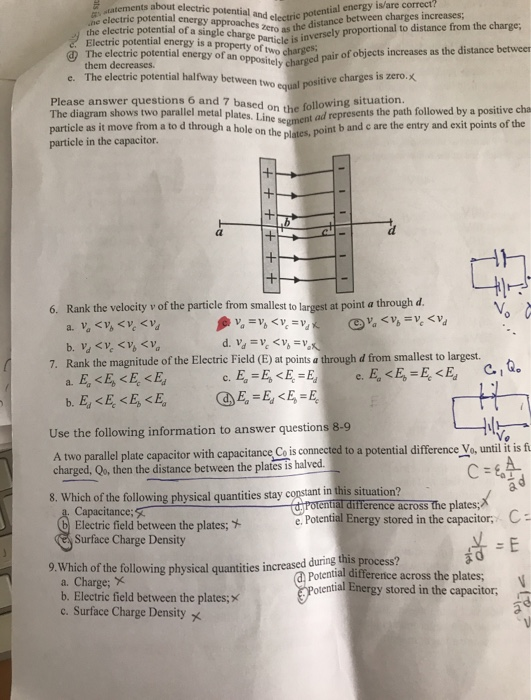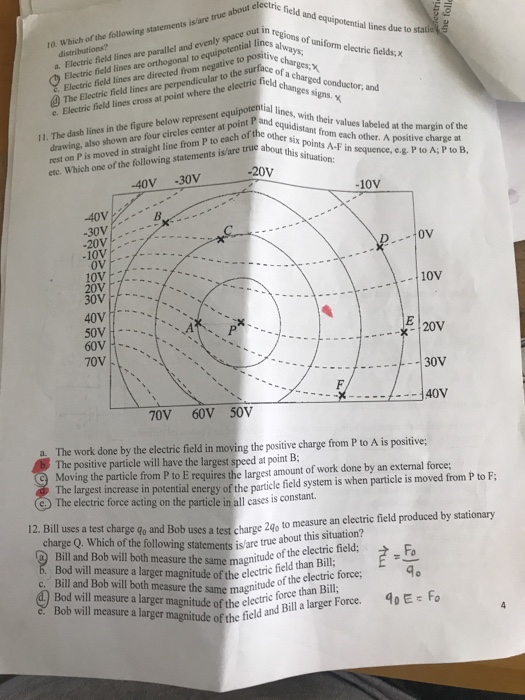astatements about electric potential and electric potential energy is/are correct? he electric potential energy approaches zero as the distance between charges increases; the electric potential of a single charge particle is inversely proportional to distance from the charge, Electric potential energy is a property of two charges The electric potential energy of an oppositely charged pair of objects increases as the distance betweem e. The electric potential halfway between two equal positive charges is zero.x them decreases. Please answer questions 6 and 7 based on the following situation. The diagram shows two parallel metal plates. Line segment ad represents the path followed by a positive ch particle as it move from a to d through a hole on the plates, point b and c are the entry and exit points of the particle in the capacitor. 6. Rank the velocity v of the particle from smallest to largest at point a through d a. ,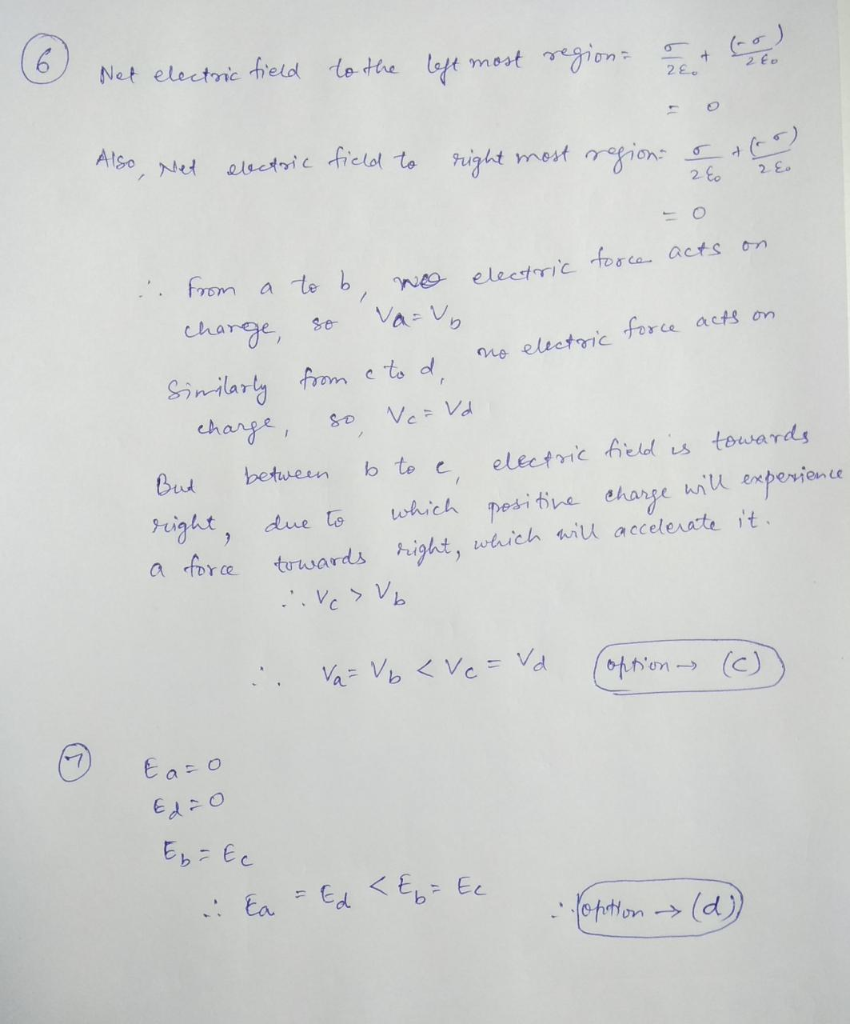#### Earn Coin

Coins can be redeemed for fabulous gifts.

Similar Homework Help Questions
• ### (a) State the units of electric potential and electric potential energy. Sketch an equipotential ...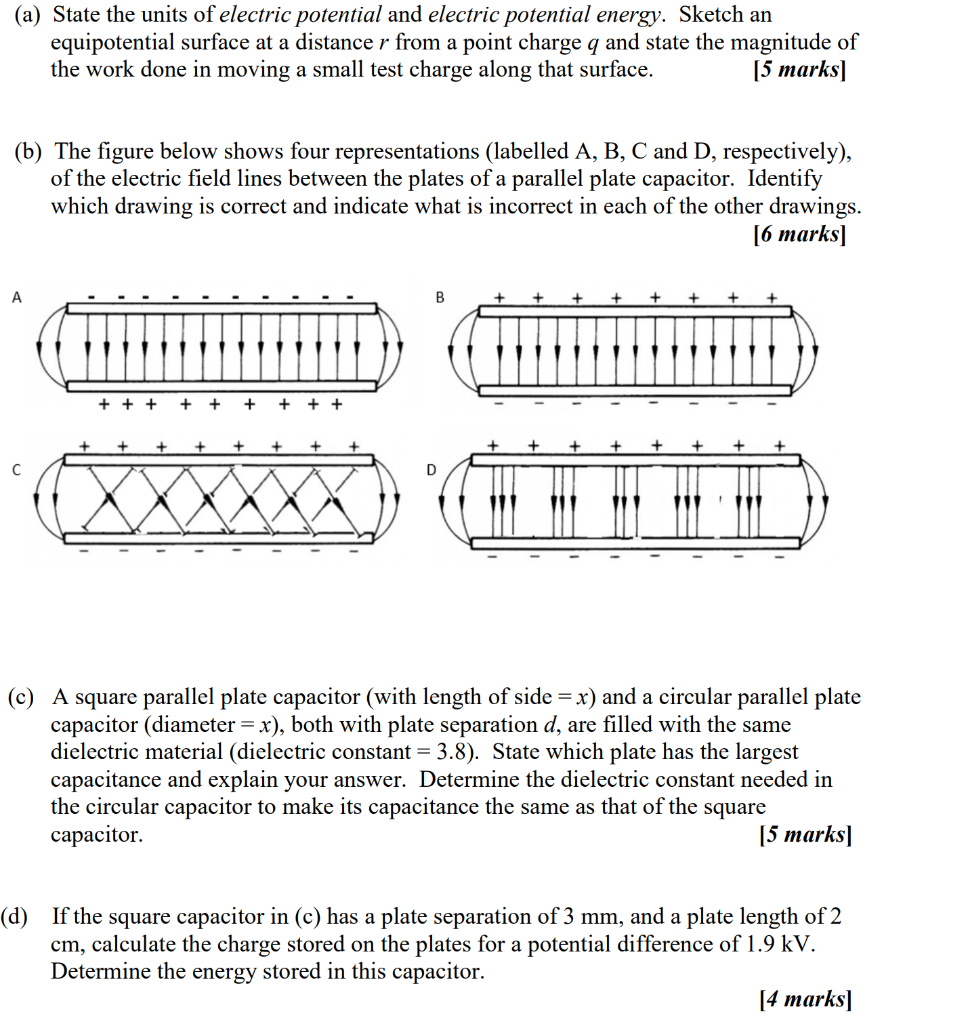(a) State the units of electric potential and electric potential energy. Sketch an equipotential surface at a distance r from a point charge q and state the magnitude of the work done in moving a small test charge along that surface. [5 marks] (b) The figure below shows four representations (labelled A, B, C and D, respectively), of the electric field lines between the plates of a parallel plate capacitor. Identify which drawing is correct and indicate what is incorrect...

• ### Page #4 Physics Il caline lab: Electric Field & Elecric Potential Part I: Electric Field Open...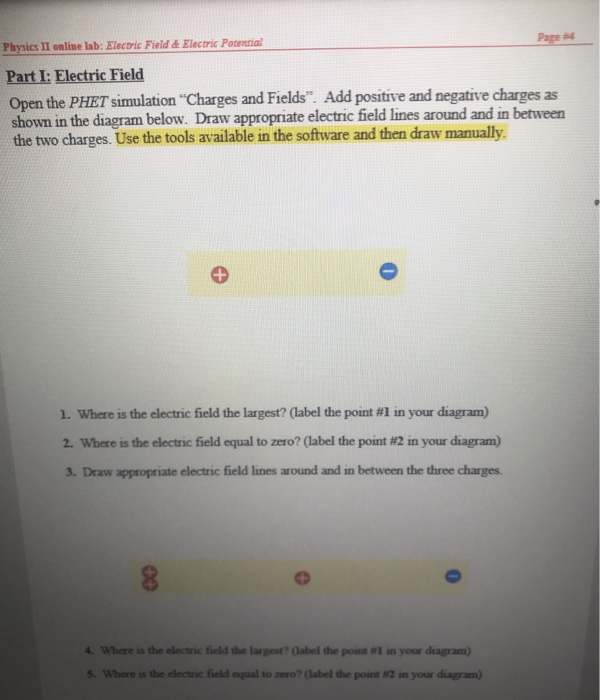Page #4 Physics Il caline lab: Electric Field & Elecric Potential Part I: Electric Field Open the PHET simulation "Charges and Fields". Add positive and negative charges as shown in the diagram below. Draw appropriate electric field lines around and in between the two charges. Use the tools available in the software and then draw manually. Where is the electric field the largest? (label the point #1 in your diagram) 2. Where is the electric field equal to zero? (label...

• ### 1. A particle with a charge of +4.20 nC is in a uniform electric field E⃗  directed...

1. A particle with a charge of +4.20 nC is in a uniform electric field E⃗  directed to the negative x direction. It is released from rest, and after it has moved 6.00 cm , its kinetic energy is found to be 1.50×10−6 J . a. What work was done by the electric force? b. What was the change in electric potential over the distance that the charge moved? c. What is the magnitude of E? d. What was the change...

• ### 3.1 Pre-lab In the lab on electric potential and electric field lines, you noted that charged...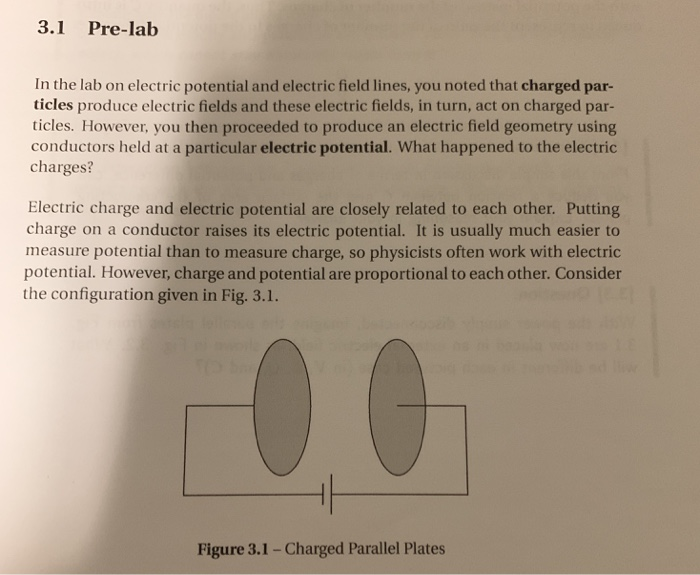3.1 Pre-lab In the lab on electric potential and electric field lines, you noted that charged par- ticles produce electric fields and these electric fields, in turn, act on charged par- ticles. However, you then proceeded to produce an electric field geometry using conductors held at a particular electric potential. What happened to the electric charges? Electric charge and electric potential are closely related to each other. Putting charge on a conductor raises its electric potential. It is usually much...

• ### Can there be a point between two equal negative charges where the electric potential is zero?...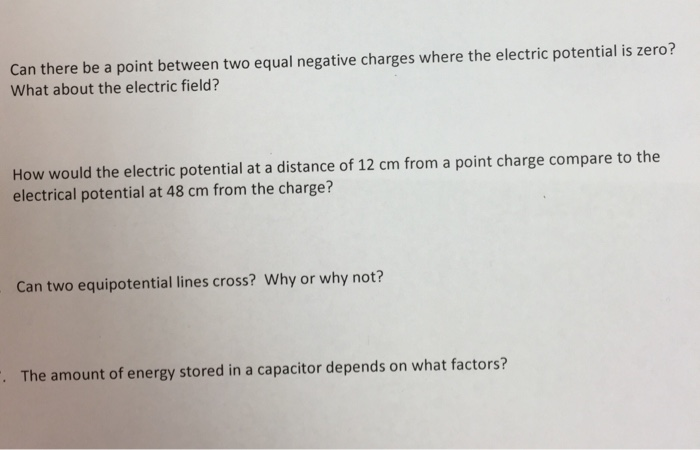Can there be a point between two equal negative charges where the electric potential is zero? What about the electric field? How would the electric potential at a distance of 12 cm from a point charge compare to the electrical potential at 48 cm from the charge? Can two equipotential lines cross? Why or why not? The amount of energy stored in a capacitor depends on what factors?

• ### WEEK 3, QUESTION 2 Which of the following is false about electric potential energy of two...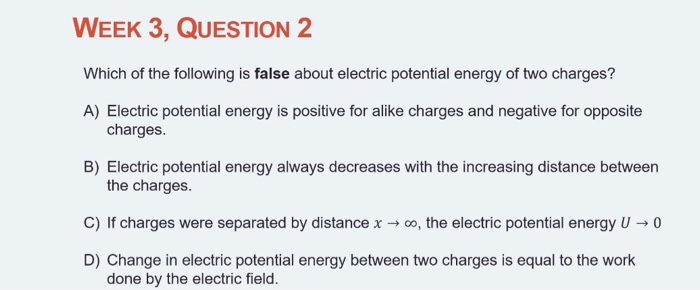WEEK 3, QUESTION 2 Which of the following is false about electric potential energy of two charges? A) Electric potential energy is positive for alike charges and negative for opposite charges. B) Electric potential energy always decreases with the increasing distance between the charges. C) If charges were separated by distance x oo, the electric potential energy U0 D) Change in electric potential energy between two charges is equal to the work done by the electric field.

• ### WEEK 3, QUESTION 2 Which of the following is false about electric potential energy of two...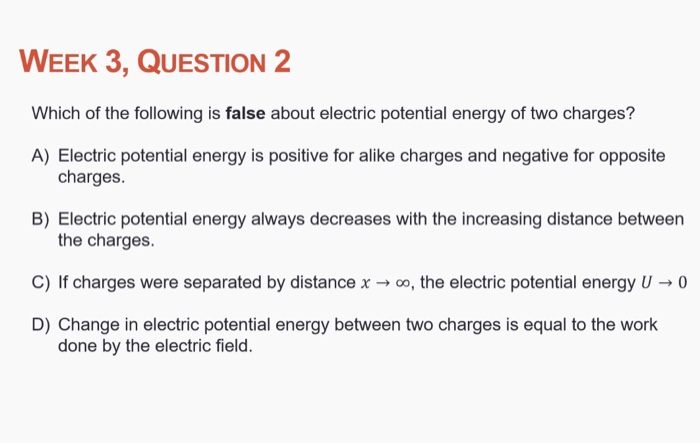WEEK 3, QUESTION 2 Which of the following is false about electric potential energy of two charges? A) Electric potential energy is positive for alike charges and negative for opposite charges. B) Electric potential energy always decreases with the increasing distance between the charges. C) If charges were separated by distance x-oo, the electric potential energy U0 D) Change in electric potential energy between two charges is equal to the work done by the electric field

• ### The potential difference between the two parallel plates shown in the drawing is 200 V. The...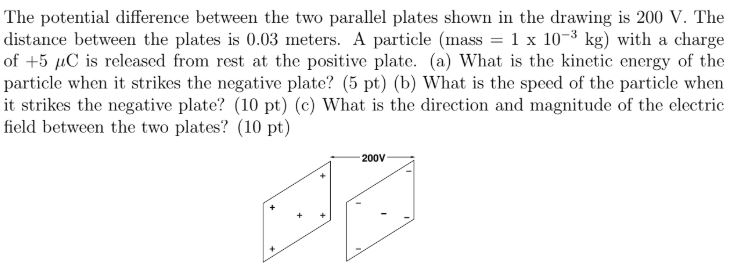The potential difference between the two parallel plates shown in the drawing is 200 V. The distance between the plates is 0.03 meters. A particle (mass = 1 x 10-3 kg) with a charge of +5 C is released from rest at the positive plate. (a) What is the kinetic energy of the particle when it strikes the negative plate? (5 pt) (b) What is the speed of the particle when it strikes the negative plate? (10 pt) (e) What...

• ### v3. At what distance from a point charge of 4.0 nC does the electric field have...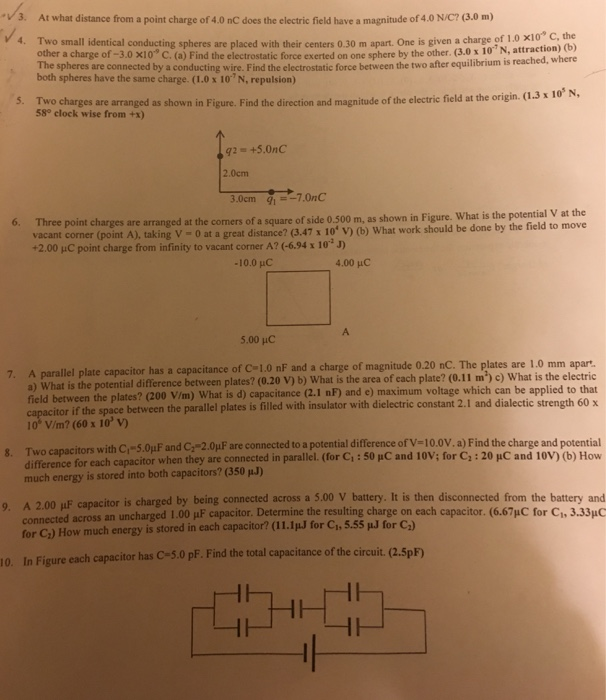v3. At what distance from a point charge of 4.0 nC does the electric field have a magnitude of 4.0 N/C? (3.0 m) 4. Two small identical conducting spheres are placed with their centers 0.30 m apart. One is given a charge of 1 0 x10* c other a charge of -3.0 x10 C. (a) Find the electrostatic force exerted on one sphere by the other. (3.0 x 10"N, attraction) the (b) e spheres are connected by a conducting wire....

• ### 5. (a) Consider an electric dipole with dipole moment p inside an external electric field E. We h...5. (a) Consider an electric dipole with dipole moment p inside an external electric field E. We have computed the potential energy U of the dipole in chapter 21. We can derive the same expression by making use of the potential concept as -9 t9 where tq are the charges that make up the dipole, F are the corresponding positions and V(F) is the potential due to all external charges. Show that, in the dipole approxi- mation (in the ii...

Free Homework App